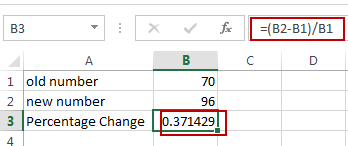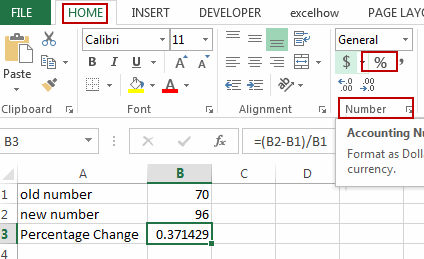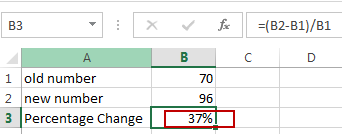# How to Calculate Percentage Change between Two Numbers in Excel

This post will guide you how to calculate percentage change between two given numbers in Excel. How do I get percentage increase or decrease between two numbers in Excel.

## Calculate Percentage Change

Assuming that you have two numbers, and one is old number, and another is new number, and you need to calculate percentage change (increase or decrease) for those two numbers in your worksheet. You can use a formula to achieve the result.  Like this:

`=(B2-B1)/B1`

Type this formula into a blank cell to place the last percentage change value, and press Enter key to apply this formula.Keep to select this cell, and go to HOME tab, click Percent icon under Number group to format the selected cell as percentage format.Sidebar